AP Chemistry : Kinetics and Energy

Example Questions

1 2 3 4 6 Next →

Example Question #1 : Reaction Energy Profile

The following diagram shows how the energy changes as the chemical reaction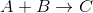progresses.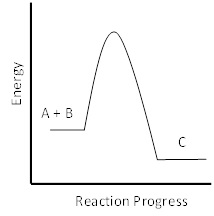A chemical engineer wants to, both, increase yield and accelerate this reaction rate. He should __________.

increase the temperature only

extractcontinuously from the reactor only

extractcontinuously from the reactor and use a catalyst

Use a catalyst only

extractcontinuously from the reactor and use a catalyst

Explanation:

"Increase the temperature" is incorrect because we have an exothermic reaction and by increasing the temperature the equilibrium constant will become smaller. An increase in temperature will shift the equilibrium toward reagents. According to the Le Chatelier's principle, by extractingcontinuously from the reactor the equilibrium shifts toward the product,. Furthermore, the use of a catalyst increases the reaction rate. The Haber process to synthesize ammonia is an example of this kind of reaction.

Example Question #2 : Reaction Energy Profile

If the activation energy of the forward reaction is greater than the activatoin energy of the reverse reaction, then this reaction is __________.

endothermic

exothermic

uncatalyzed

spontaneous

endothermic

Explanation:

If the activation energy of a forward reaction is greater than that of the reverse reaction, thenthe products must have a higher enthalpy than the reactants (draw a potential energy diagram to visualize).

Example Question #1 : Reaction Energy Profile

If the activation energy of a forward reaction is greater than the activation energy of a reverse reaction, what must be true of the reaction?

It is nonspontaneous

It is at equilibrium

It is exothermic

It is endothermic

It is spontaneous

It is endothermic

Explanation:

If the activation energy of the forward reaction is greater than that of the reverse reaction, the products must have a higher enthalpy than the reactants. The net enthalpy change is therefore positive, meaning that it is endothermic.

Example Question #1 : Reaction Energy Profile

Which of the following is true regarding activation energy?

I. Activation energy depends on the pressure of the system

II. The reaction rate decreases as the activation energy increases

III. Catalysts increase the activation energy of a reaction

I only

I and III

II only

I and II

II only

Explanation:

Activation energy is the energy barrier that needs to be overcome for a reaction to proceed; the higher the activation energy, the slower the reaction. Activation energy can only be altered via a catalyst. Catalysts are chemical substances that lower the activation energy, allowing reactions to proceed faster. Other physical quantities such as temperature and pressure don’t alter the activation energy.

Reaction rate is a direct measure of the speed of a reaction. As mentioned, increasing activation energy will increase the barrier and, therefore, slow down the reaction.

Catalysts are chemical substances that decrease the activation energy, thereby increasing the reaction rate. They are commonly used in chemical reactions to drastically speed up reactions that might otherwise take hours or days to complete. Enzymes are biological catalysts that facilitate most of the biological reactions happening in our bodies.

Example Question #5 : Reaction Energy Profile

__________ the activation energy of a reaction will __________ the amount of products produced.

Increasing . . . increase

Decreasing . . . decrease

Increasing . . . not change

Decreasing . . . increase

Increasing . . . not change

Explanation:

This question is asking about the relationship between activation energy of a reaction (kinetics) and the amount of products produced (equilibrium). Remember that altering the speed of a reaction (kinetics) does not change the equilibrium of the reaction. Increasing or decreasing activation energy (which alters the speed of reaction) will simply allow for the reaction to proceed slower or faster, respectively. It will not change the amount of products produced at the end.

Example Question #2 : Reaction Energy Profile

Which of the following is the correct way to calculate the activation energy of a reaction?

Energy of reactant – Energy of transition state = Activation energy

Energy of transition state – Energy of product = Activation energy

Energy of transition state – Energy of reactant = Activation energy

Energy of product – Energy of transition state = Activation energy

Energy of transition state – Energy of reactant = Activation energy

Explanation:

Activation energy is the difference between the energy of the transition state and the energy of the reactant. Recall that activation energy is the energy barrier that needs to be overcome by a reaction. The transition state is a higher-energy, intermediary molecule that lies in between the reactants and products. It is created when reactants are in the process of becoming products. Therefore, to get to products, it is necessary to go through this high-energy transition state.

Activation energy is the energy needed to climb this energy “hill” (energy needed to go from reactants to transition state); therefore, the activation energy is the energy of the transition state (top of the energy hill/barrier) minus the energy of the reactant.

Example Question #7 : Reaction Energy Profile

Consider the following reaction: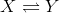The conversion ofto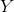releases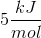of energy and has an activation energy of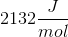.

What is the activation energy of the reverse reaction?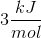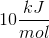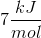Cannot be determined from the given informationExplanation:

To answer this question, we have to consider an energy diagram for an exothermic reaction. We know the forward reaction is exothermic because the question states that energy is released. We are given the activation energy of the reaction (the energy difference between the activated complex, or transition state, and the reactants) and the energy released (the energy difference between the products and the reactants). In the reverse reaction we will be going from products to reactants as follows: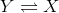This means that the activation energy for reverse reaction will be higher (has to climb a higher hill from “products” to “activated complex”). Using the given information we can deduce that the activation energy (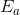) of the reverse reaction is the SUM of the activation energy of the forward reaction AND the energy released from the forward reaction.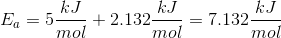The closest answer is. Note that the forward reaction is exothermic, whereas the reverse reaction is endothermic (energy is being consumed). The reverse reaction is endothermic because the reactant () has lower energy than the product ().

Example Question #8 : Reaction Energy Profile

Which of the following is true regarding activation energy?

Increasing the temperature will decrease the activation energy

A catalyst increases the energy of the reactants

Enthalpy of a reaction has no effect on the activation energy

Activation energy determines the equilibrium constant of a reaction